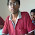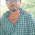# Pharma Engineering

An Engineer's Choice

• ## Thursday, 11 June 2015ORDER OF REACTION
Order:

This term can be defined as the sum of the powers of the concentrations of the reactants in rate expression. This is what every student know theoretically,
-ra =kCa2Cb
So whats the order now,
every one answers the order is 3,
but when a student enters the professional large scale manufacturing industries, the one should need to apply the theory to the practical cases, but I guarantee you that whatever you studied in the text books is quite different from the practical, as the majority of the problems solved on paper in the engineering classes consists of a common line, “NEGLECT THE LOSSES”, which makes a huge difference, so today lets start to learn how to apply the theory we studied in our books to the large scale, and determine the ORDER OF REACTION.

PRACTICAL  APPROACH  TO  DETERMINE  ORDER  OF  REACTION:

Method 1:
Case study:
Consider a reaction where two components reacts in a solvent medium, for example , the large scale process is,
1)      Charge methanol 2000lts into reactor.
2)      Charge 200kgs of citric acid(X) into reactor, and stir for 25(+/-)5minutes.
3)      Charge 500kgs of Soda Ash(Y) into the reactor.
4)      Maintain the Mass temp 50(+/-)5 for 5hrs(+/-)1hr.
5)      ……..
6)      ……………..
7)      ………………………..
8)      ……………………………………….
9)      …………………………………………………
10)   Unload the residue mass into containers and weigh it, the required product(P)  yield must be 600(+/-)50kgs
So, now this is the case and now first find the KSM(key starting material) , and then what are the reactants, as we here charging methanol, citric acid and soda ash, all the three were not reactants, methanol is just used to provide a medium for reaction, so now the main reactants were citric acid and soda ash,
So to find the order of reaction, just take the each reactant, first lets take citric acid, 200kgs used so find the number of moles,
Number of moles of citric acid= 200/( Mol. Wt. of citric acid) =a say
Number of moles of Soda ash= 500/( Mol. Wt. of Soda ash) = b say.
Now move to the yield we got  i.e., 600kgs, so now find the Product moles,
Number of moles of Product, =600/(Mol. Wt. of Product) =c say
Now write the reaction,  aX +bY  à cP ,
Now find number of moles of citric acid required to produce one mole of product, i.e.,
K=(a/c)*(minimum number of moles of citric acid required to form the product).
Find number of moles of soda ash required to produce one mole of product, i.e.,
L=( b/c)*(minimum number of moles of soda ash required to form product).
So , now write the rate expression,

rP  = kCXKCYL
Now find the order, it will be a/c + b/c= (a+b)/c.
Method 2:
Consider a reaction where two components reacts in a solvent medium, for example , the large scale process is,
1)      Charge methanol 2000lts into reactor.
2)      Charge 200kgs of citric acid(X) into reactor, and stir for 25(+/-)5minutes.
3)      Charge 500kgs of Soda Ash(Y) into the reactor.
4)      Maintain the Mass temp 50(+/-)5 for 5hrs(+/-)1hr.
5)      ……..
6)      ……………..
7)      ………………………..
8)      ……………………………………….
9)      …………………………………………………
10)           Unload the residue mass into containers and weigh it, the required product(P)  yield must be 600(+/-)50kgs
Theoretical combined formula for calculating the order/reaction time/rate constant is,
(1/Ca)(1-n) –(1/Ca0)(1-n) =k(n-1)t

Ca  refers to the final concentration of citric acid, and Ca0  refers to the initial concentration of citric acid, n refers to the order of reaction, k refers to the rate constant, and t refers to the reaction time,
So now to find the order from the above equation we need the rate constant and reaction time, we can get the initial concentration and final concentration from the HPLC/GC reports.
In our case study, its mentioned that the maintainence time is 5hrs, so the sampling to find the Ca, Cao must be done before and after of the maintainence time, and the amount of KSM % i.e., Citric acid percent gives us Ca, Cao. And whenever its mentioned Maintainence time in the procedure, it will be the critical parameter in the process and it will be the reaction time, so now the reaction time is 5hrs.
So now we have to find the rate constant , rate constant characterizes the conversion, means amount of KSM decomposed / disappeared in the particular reaction time, so now lets say 50% decomposed in a whole reaction time of 5 hrs then it will be 200*(50/100) =100kgs. So 100 kgs decomposed in 5 hrs, so Rate constant k will be
i.e.,
=(100/Mol. Wt. of KSM)/ (vol of Reactants*time),
Now substitute and solve the eqn (1/Ca)(1-n) –(1/Ca0)(1-n) =k(n-1)t

for order. That’s it, if you understood it, then say cheers and if you have any queries feel free to comment, sharing is caring, I care for youHi! I am Ajay Kumar Kalva, Currently serving as the CEO of this site, a tech geek by passion, and a chemical process engineer by profession, i'm interested in writing articles regarding technology, hacking and pharma technology.

1.how to design reator??

2.how to design batch reactor?

1.Dear Nitin,

Designing a batch reactor depends upon our requirement, from process requirement to available area where we need to install the reactor, every minute thing shall be evaluated and then they need to be considered for designing.
Coming to agitator, the energy dissipation need to be evaluated based on process requirement, then based on that power no. shall be calculated, then the agitator shall be selected,
Based on the agitator, the motor & gearbox shall be selected,
Based on the heat duty of the reactor jacket shall be selected, whether annular/limpet/double limpet coil.

Regards,
AJAY K

3.How can find the rate of reaction and what is the meaning of munus symble (-r) in rate equation.

1.Dear Digamber,

Rate of reaction can be practically found through chromatography techniques.

Best Regards,
AJAY K

4.What purpose rate of reaction main uses

1.Rate of reaction indicates the conversion rate, conversion of raw materials and it can be helpful to decide/fix the reaction time, based on the reaction rate and through effective scale-up the capacity can be calculated in plant scale.

Best Regards,
AJAY K

5.Hi Sir
I want know calculation for steam flow at given pressure through line.
What will be steam flow at 2kg/cm2 pressure inlet to reactor coil.
Regards
PANKAJ CHAUDHARY

1.Hiii Pankaj,

Use the relation for flowrate: Ms = 11304 x (v/V) x (r^2).

v - Steam velocity(m/s),
Ms - Mass flow rate (Kg/hr),
V - Specific volume (Cu.m/Kg).

** Pressure & Flow rate are not related.Hi! I am Ajay Kumar Kalva, Currently serving as the CEO of this site, a tech geek by passion, and a chemical process engineer by profession, i'm interested in writing articles regarding technology, hacking and pharma technology.